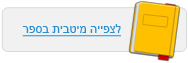עמוד:36 Activity Interactive Simulation Energy of a Pendulum Run the simulation , Energy of a Pendulum . Check the friction box , run the simulation and answer the questions below . Questions 1 . Which types of energy decrease in quantity over time ? How is this seen in the chart ? 2 . Which type of energy increases constantly ? How is this seen in the chart ? 3 . What remains constant ? How is this seen in the chart ? 4 . Explain , based on the simulation , why the pendulum finally comes to a stop and why this does not contradict the law of conservation of energy ?© מטח - המרכז לטכנולוגיה חינוכית